|

# 轻松解决厨房难题 箭牌美式田园“新枫叶情”橱柜评测

有些朋友不喜欢下厨，宁愿叫外卖或出去吃，觉得省事多了。但时间一长你也会腻，外面煮的不如自己煮的卫生干净，而且一家人在家里吃饭也倍儿温馨。然而当你一进厨房，不时把厨房弄得乱七八糟，还要收拾烂摊子，十分麻烦。一个好的橱柜就能帮你轻松解决厨房难题，规划合理的厨房分区让你轻松下厨，强大的收纳既让你随时能找到所需物品，又能让厨房保持整洁干净，设计好看的橱柜也让你在客人面前更有面子。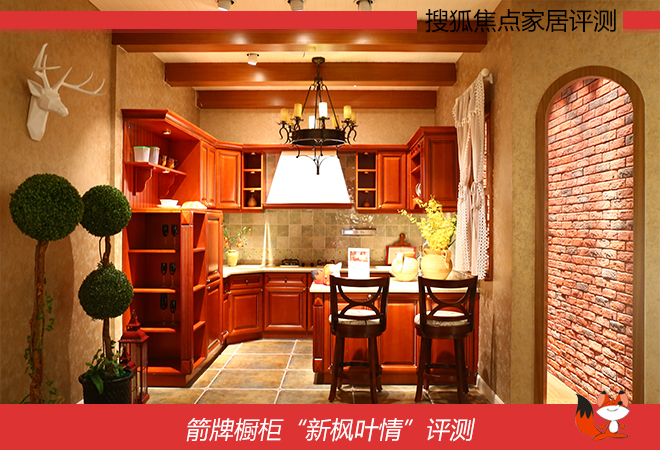今天搜狐焦点家居评测室为大家推荐的正是这样的好橱柜，它就是箭牌橱柜“新枫叶情”。“新枫叶情”橱柜到底有什么魅力呢？我们一起来看看吧！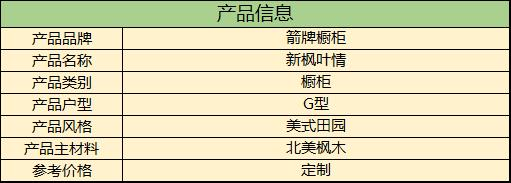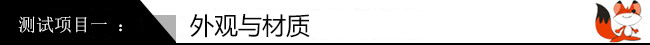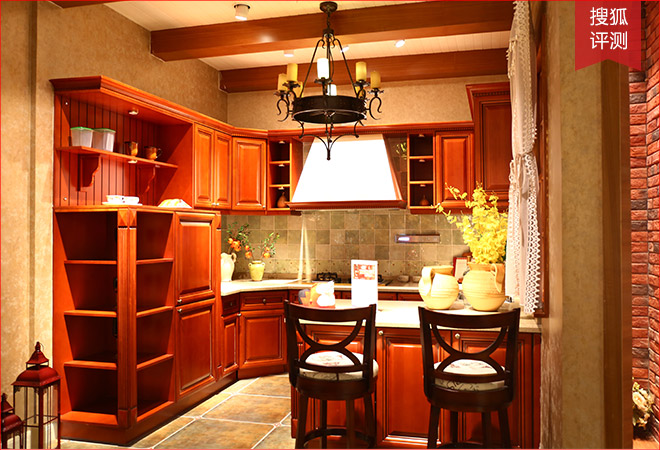箭牌的新枫叶情橱柜，线条简洁又利落，主体是不会过分沉重的红木色，既有开放式空间，也有封闭式柜体，交相呼应，形成凹凸有致的结构，给人一种轻重交错平衡的视觉效果，尽显美式田园的自然亲切、实用简洁。另外，新枫叶情是G型设计，适用于相对大而宽敞的厨房空间，划分了厨房的每个功能分区，提高了工作效率，又增加了收纳空间，适合较多成员或对收纳需求大的家庭。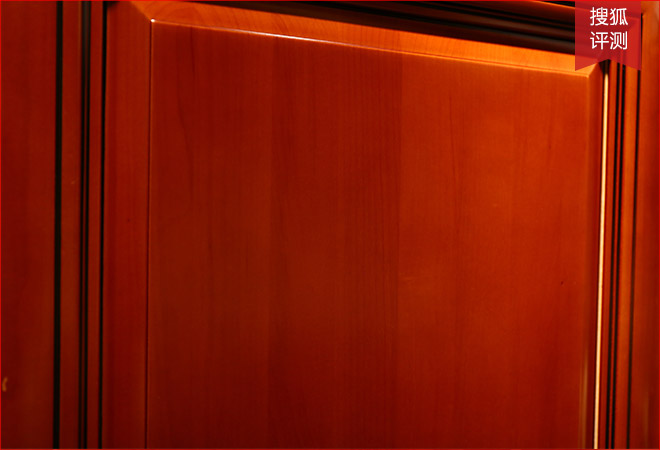这款产品采用进口的北美枫木，纹理细腻清晰，触感温柔，最实在的原木色带来一股清新舒适、恬静休闲的田园气息。再加上箭牌橱柜采用了德国豪迈技术封边，橱柜的边缘十分光滑，因此它不易受潮变形，经久耐用。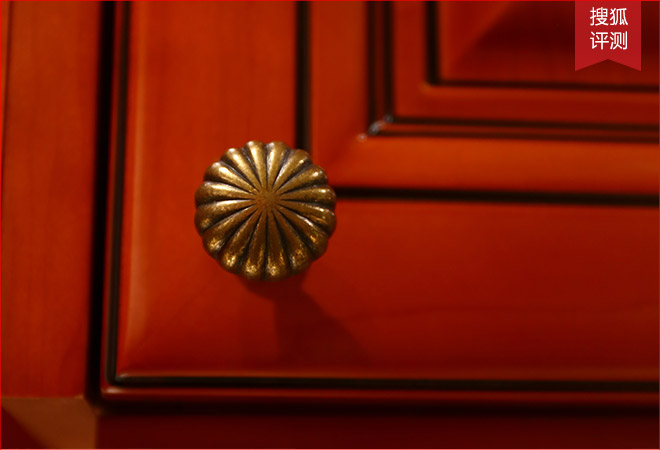仿古模样的拉手，泛着淡淡的金色光泽，配上最简单的弧形线条雕刻在上面，这样的细节更显美式田园风格的简朴自然。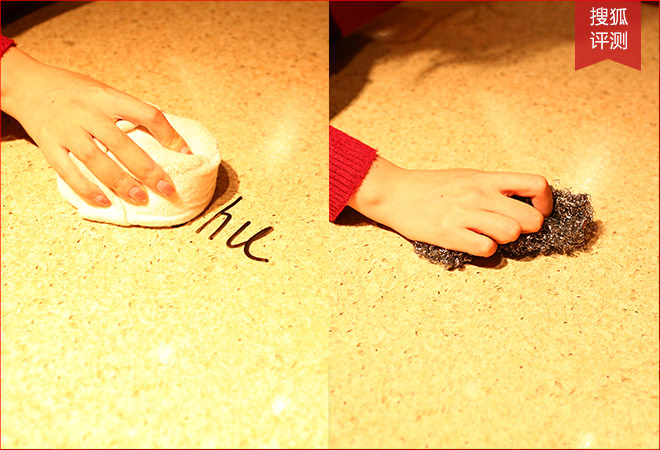台面是石英石材质，可与食物直接接触，更采用了先进的纳米技术，具有超耐磨、抗高温、耐腐蚀等优异性能。我们用油性笔在台面上写字，稍等片刻后即可轻松擦除，还用钢丝擦来回摩擦台面。经过这两种测试后，我们发现台面依然光亮如新，说明台面的抗污性与耐磨性十分强。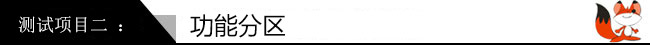清洗区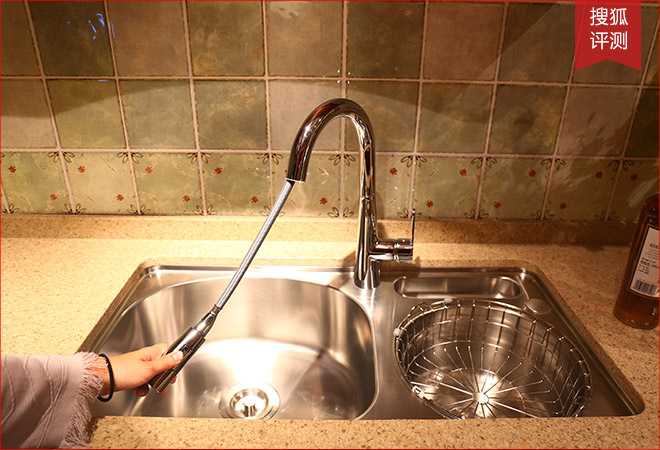箭牌新枫叶情橱柜的水槽，采用食品级SUS304不锈钢，耐用耐磨，安全使用。水槽分为三个板块，有蔬果清洗区、餐具清洗区、残渣存放区，满足最基本的使用需求。值得留意的是，它的龙头可以拉伸使用，有两种出水模式随意选择。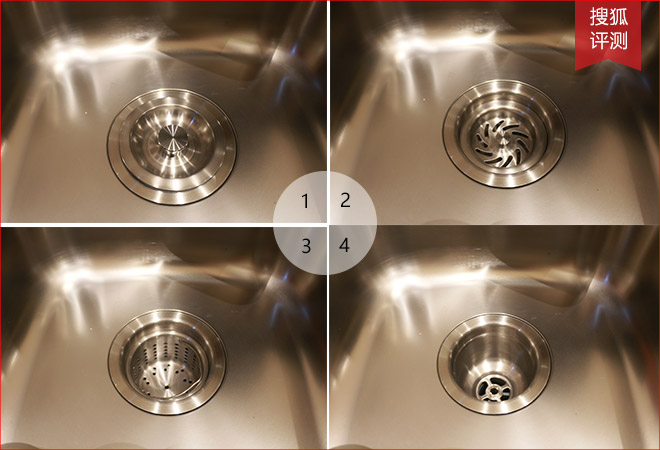去水口，有多层金属配件隔离水、残渣等其他杂质，清洁方便。

收纳区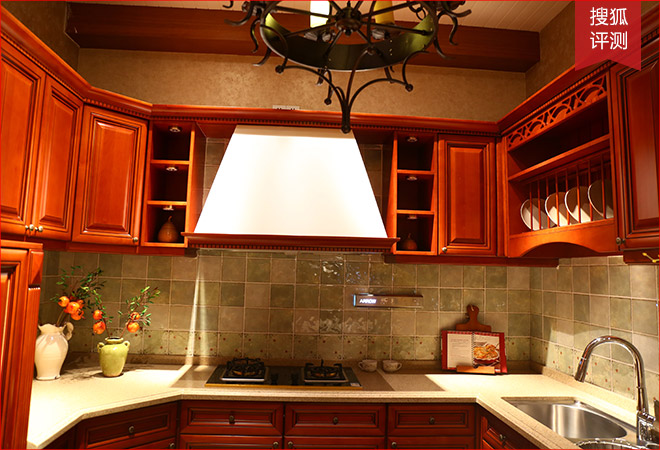箭牌新枫叶情橱柜有多个收纳区，既有开放式的储物柜可以摆放碗碟等展示，也有封闭式大小不一的储物柜，满足各种厨具和食品的摆放，让厨房简洁干净。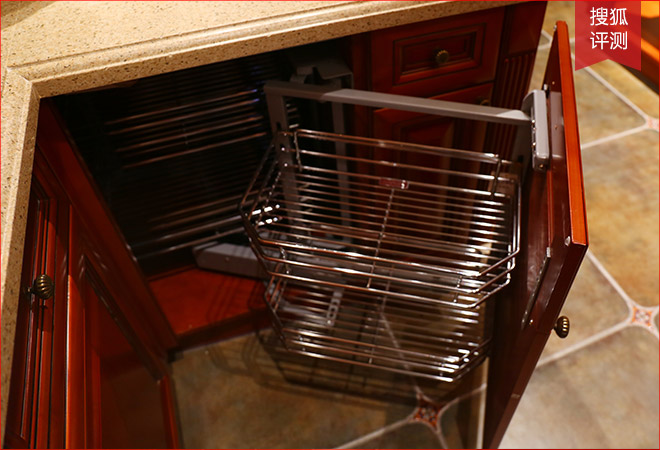新枫叶情橱柜更准备了拉篮，能将厨具餐具分放在网架中，方便整洁。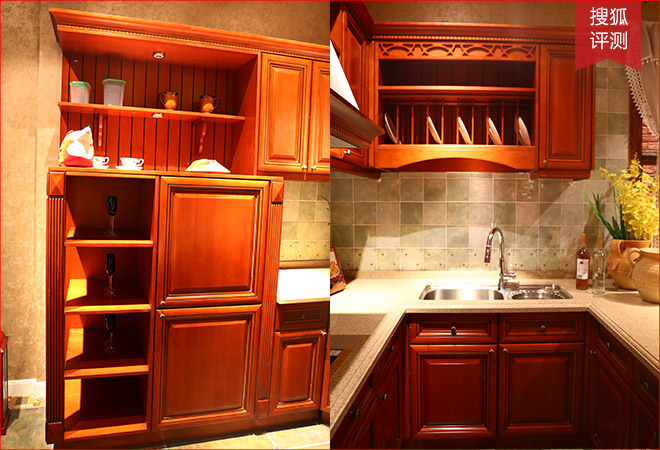最左侧的柜子，如左图，有开放空间可以摆放红酒、酒杯。而如右图的水槽，下面还有储物空间，充分利用空间，也采用了优质食品级SUS304不锈钢，有效隔离水渍，防止柜身板发胀。

烹饪区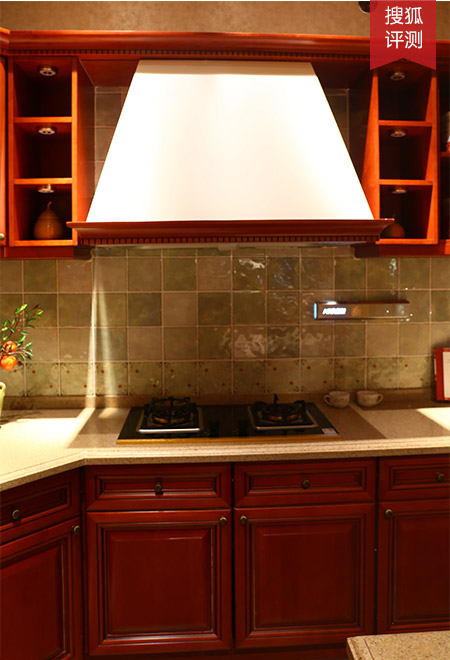新枫叶情橱柜的烹饪区在中间位置，上方是吸力强劲、节能环保的烟机，下方是不锈钢的炉灶，全铜火盖，0秒点火启动精准，无论是烟机还是炉灶，都被箭牌橱柜完美的融入到整体橱柜中。烟机的纯白、炉灶的银色与柜体颜色形成鲜明的对比，却又十分协调。

休闲区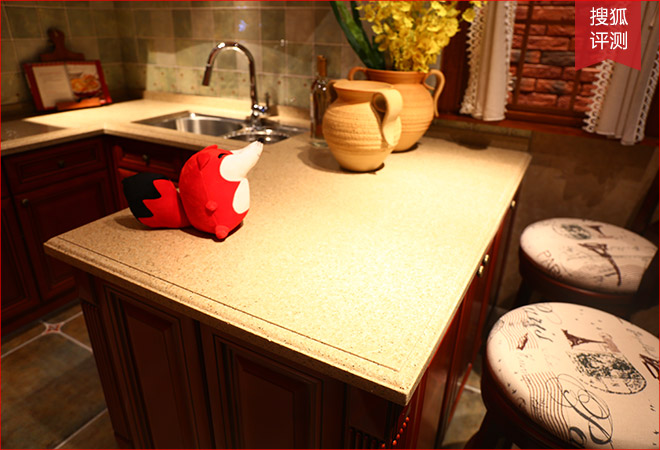新枫叶情橱柜更有休闲区兼餐厅区，我们可以摆放新鲜的蔬菜肉类，也可以在这里直接用餐，橱柜的功能十分强大。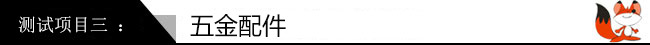五金配件虽然是配角，但却影响着橱柜的使用寿命。而箭牌橱柜的五金配件，表面经过5层多金属复合电镀，形成了强而有效的保护层，不锈不腐，光洁亮丽。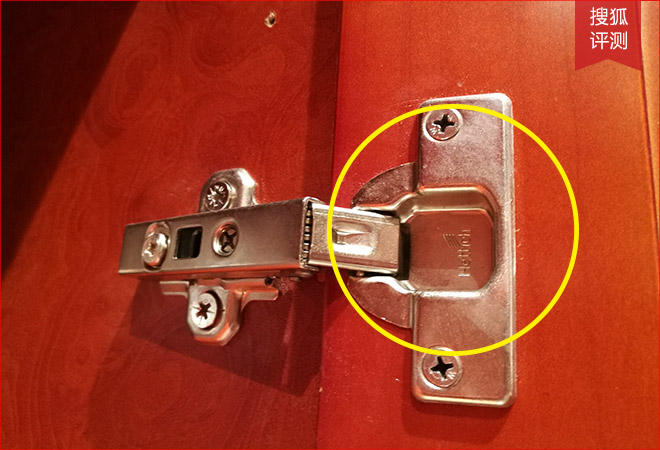新枫叶情橱柜使用德国海蒂诗五金，有15万次开关无障碍、25度角自动闭合以及快捷装卸等众多优点，安全便捷。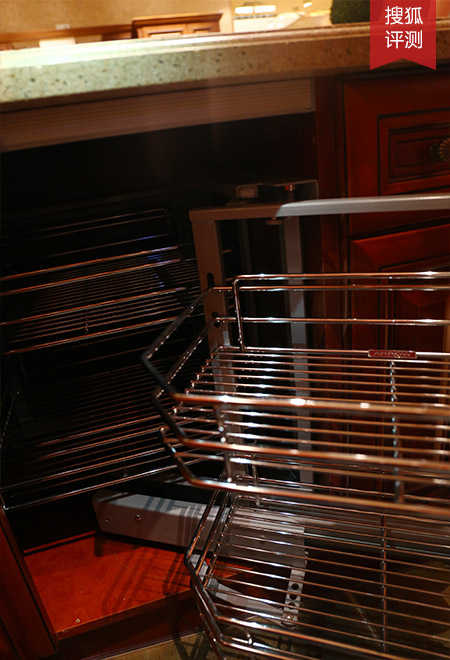我们尝试开合几次拉篮，发现没有刺耳声音发出，十分安静。这是因为箭牌橱柜使用了优质导轨，更顺畅，更安静，闭合更紧密，有效避免摩擦噪音。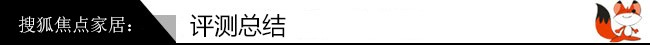箭牌新枫叶情橱柜，美式田园风格，流畅的线条展现力量感，细节与结构营造一种轻重交错的视觉感受。主材质是北美枫木，尽显原木色彩与纹理，扑面而来的是美式田园的清新舒适，五金配件均有品牌保证，经久耐用。 橱柜为G型设计，划分了厨房的清洗、收纳、烹饪、休闲多个功能分区，提高了工作效率，又增加了收纳空间。箭牌新枫叶情橱柜，适合宽敞的厨房空间，或有较多成员，或对收纳需求大的家庭。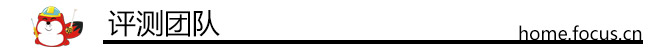拍摄&文字：郭志华

`声明：本文由入驻焦点开放平台的作者撰写，除焦点官方账号外，观点仅代表作者本人，不代表焦点立场错误信息举报电话： 400-099-0099，邮箱：jubao@vip.sohu.com，或点此进行意见反馈，或点此进行举报投诉。`A B C D E F G H J K L M N P Q R S T W X Y Z
A - B - C - D - E
• A
• 鞍山
• 安庆
• 安阳
• 安顺
• 安康
• 澳门
• B
• 北京
• 保定
• 包头
• 巴彦淖尔
• 本溪
• 蚌埠
• 亳州
• 滨州
• 北海
• 百色
• 巴中
• 毕节
• 保山
• 宝鸡
• 白银
• 巴州
• C
• 承德
• 沧州
• 长治
• 赤峰
• 朝阳
• 长春
• 常州
• 滁州
• 池州
• 长沙
• 常德
• 郴州
• 潮州
• 崇左
• 重庆
• 成都
• 楚雄
• 昌都
• 慈溪
• 常熟
• D
• 大同
• 大连
• 丹东
• 大庆
• 东营
• 德州
• 东莞
• 德阳
• 达州
• 大理
• 德宏
• 定西
• 儋州
• 东平
• E
• 鄂尔多斯
• 鄂州
• 恩施
F - G - H - I - J
• F
• 抚顺
• 阜新
• 阜阳
• 福州
• 抚州
• 佛山
• 防城港
• G
• 赣州
• 广州
• 桂林
• 贵港
• 广元
• 广安
• 贵阳
• 固原
• H
• 邯郸
• 衡水
• 呼和浩特
• 呼伦贝尔
• 葫芦岛
• 哈尔滨
• 黑河
• 淮安
• 杭州
• 湖州
• 合肥
• 淮南
• 淮北
• 黄山
• 菏泽
• 鹤壁
• 黄石
• 黄冈
• 衡阳
• 怀化
• 惠州
• 河源
• 贺州
• 河池
• 海口
• 红河
• 汉中
• 海东
• I
• J
• 晋中
• 锦州
• 吉林
• 鸡西
• 佳木斯
• 嘉兴
• 金华
• 景德镇
• 九江
• 吉安
• 济南
• 济宁
• 焦作
• 荆门
• 荆州
• 江门
• 揭阳
• 金昌
• 酒泉
• 嘉峪关
K - L - M - N - P
• K
• 开封
• 昆明
• 昆山
• L
• 廊坊
• 临汾
• 辽阳
• 连云港
• 丽水
• 六安
• 龙岩
• 莱芜
• 临沂
• 聊城
• 洛阳
• 漯河
• 娄底
• 柳州
• 来宾
• 泸州
• 乐山
• 六盘水
• 丽江
• 临沧
• 拉萨
• 林芝
• 兰州
• 陇南
• M
• 牡丹江
• 马鞍山
• 茂名
• 梅州
• 绵阳
• 眉山
• N
• 南京
• 南通
• 宁波
• 南平
• 宁德
• 南昌
• 南阳
• 南宁
• 内江
• 南充
• P
• 盘锦
• 莆田
• 平顶山
• 濮阳
• 攀枝花
• 普洱
• 平凉
Q - R - S - T - W
• Q
• 秦皇岛
• 齐齐哈尔
• 衢州
• 泉州
• 青岛
• 清远
• 钦州
• 黔南
• 曲靖
• 庆阳
• R
• 日照
• 日喀则
• S
• 石家庄
• 沈阳
• 双鸭山
• 绥化
• 上海
• 苏州
• 宿迁
• 绍兴
• 宿州
• 三明
• 上饶
• 三门峡
• 商丘
• 十堰
• 随州
• 邵阳
• 韶关
• 深圳
• 汕头
• 汕尾
• 三亚
• 三沙
• 遂宁
• 山南
• 商洛
• 石嘴山
• T
• 天津
• 唐山
• 太原
• 通辽
• 铁岭
• 泰州
• 台州
• 铜陵
• 泰安
• 铜仁
• 铜川
• 天水
• 天门
• W
• 乌海
• 乌兰察布
• 无锡
• 温州
• 芜湖
• 潍坊
• 威海
• 武汉
• 梧州
• 渭南
• 武威
• 吴忠
• 乌鲁木齐
X - Y - Z
• X
• 邢台
• 徐州
• 宣城
• 厦门
• 新乡
• 许昌
• 信阳
• 襄阳
• 孝感
• 咸宁
• 湘潭
• 湘西
• 西双版纳
• 西安
• 咸阳
• 西宁
• 仙桃
• 西昌
• Y
• 运城
• 营口
• 盐城
• 扬州
• 鹰潭
• 宜春
• 烟台
• 宜昌
• 岳阳
• 益阳
• 永州
• 阳江
• 云浮
• 玉林
• 宜宾
• 雅安
• 玉溪
• 延安
• 榆林
• 银川
• Z
• 张家口
• 镇江
• 舟山
• 漳州
• 淄博
• 枣庄
• 郑州
• 周口
• 驻马店
• 株洲
• 张家界
• 珠海
• 湛江
• 肇庆
• 中山
• 自贡
• 资阳
• 遵义
• 昭通
• 张掖
• 中卫

1室1厅1厨1卫1阳台

1
2
3
4
5

0
1
2

1

1

0
1
2
3报名成功，资料已提交审核A B C D E F G H J K L M N P Q R S T W X Y Z
A - B - C - D - E
• A
• 鞍山
• 安庆
• 安阳
• 安顺
• 安康
• 澳门
• B
• 北京
• 保定
• 包头
• 巴彦淖尔
• 本溪
• 蚌埠
• 亳州
• 滨州
• 北海
• 百色
• 巴中
• 毕节
• 保山
• 宝鸡
• 白银
• 巴州
• C
• 承德
• 沧州
• 长治
• 赤峰
• 朝阳
• 长春
• 常州
• 滁州
• 池州
• 长沙
• 常德
• 郴州
• 潮州
• 崇左
• 重庆
• 成都
• 楚雄
• 昌都
• 慈溪
• 常熟
• D
• 大同
• 大连
• 丹东
• 大庆
• 东营
• 德州
• 东莞
• 德阳
• 达州
• 大理
• 德宏
• 定西
• 儋州
• 东平
• E
• 鄂尔多斯
• 鄂州
• 恩施
F - G - H - I - J
• F
• 抚顺
• 阜新
• 阜阳
• 福州
• 抚州
• 佛山
• 防城港
• G
• 赣州
• 广州
• 桂林
• 贵港
• 广元
• 广安
• 贵阳
• 固原
• H
• 邯郸
• 衡水
• 呼和浩特
• 呼伦贝尔
• 葫芦岛
• 哈尔滨
• 黑河
• 淮安
• 杭州
• 湖州
• 合肥
• 淮南
• 淮北
• 黄山
• 菏泽
• 鹤壁
• 黄石
• 黄冈
• 衡阳
• 怀化
• 惠州
• 河源
• 贺州
• 河池
• 海口
• 红河
• 汉中
• 海东
• I
• J
• 晋中
• 锦州
• 吉林
• 鸡西
• 佳木斯
• 嘉兴
• 金华
• 景德镇
• 九江
• 吉安
• 济南
• 济宁
• 焦作
• 荆门
• 荆州
• 江门
• 揭阳
• 金昌
• 酒泉
• 嘉峪关
K - L - M - N - P
• K
• 开封
• 昆明
• 昆山
• L
• 廊坊
• 临汾
• 辽阳
• 连云港
• 丽水
• 六安
• 龙岩
• 莱芜
• 临沂
• 聊城
• 洛阳
• 漯河
• 娄底
• 柳州
• 来宾
• 泸州
• 乐山
• 六盘水
• 丽江
• 临沧
• 拉萨
• 林芝
• 兰州
• 陇南
• M
• 牡丹江
• 马鞍山
• 茂名
• 梅州
• 绵阳
• 眉山
• N
• 南京
• 南通
• 宁波
• 南平
• 宁德
• 南昌
• 南阳
• 南宁
• 内江
• 南充
• P
• 盘锦
• 莆田
• 平顶山
• 濮阳
• 攀枝花
• 普洱
• 平凉
Q - R - S - T - W
• Q
• 秦皇岛
• 齐齐哈尔
• 衢州
• 泉州
• 青岛
• 清远
• 钦州
• 黔南
• 曲靖
• 庆阳
• R
• 日照
• 日喀则
• S
• 石家庄
• 沈阳
• 双鸭山
• 绥化
• 上海
• 苏州
• 宿迁
• 绍兴
• 宿州
• 三明
• 上饶
• 三门峡
• 商丘
• 十堰
• 随州
• 邵阳
• 韶关
• 深圳
• 汕头
• 汕尾
• 三亚
• 三沙
• 遂宁
• 山南
• 商洛
• 石嘴山
• T
• 天津
• 唐山
• 太原
• 通辽
• 铁岭
• 泰州
• 台州
• 铜陵
• 泰安
• 铜仁
• 铜川
• 天水
• 天门
• W
• 乌海
• 乌兰察布
• 无锡
• 温州
• 芜湖
• 潍坊
• 威海
• 武汉
• 梧州
• 渭南
• 武威
• 吴忠
• 乌鲁木齐
X - Y - Z
• X
• 邢台
• 徐州
• 宣城
• 厦门
• 新乡
• 许昌
• 信阳
• 襄阳
• 孝感
• 咸宁
• 湘潭
• 湘西
• 西双版纳
• 西安
• 咸阳
• 西宁
• 仙桃
• 西昌
• Y
• 运城
• 营口
• 盐城
• 扬州
• 鹰潭
• 宜春
• 烟台
• 宜昌
• 岳阳
• 益阳
• 永州
• 阳江
• 云浮
• 玉林
• 宜宾
• 雅安
• 玉溪
• 延安
• 榆林
• 银川
• Z
• 张家口
• 镇江
• 舟山
• 漳州
• 淄博
• 枣庄
• 郑州
• 周口
• 驻马店
• 株洲
• 张家界
• 珠海
• 湛江
• 肇庆
• 中山
• 自贡
• 资阳
• 遵义
• 昭通
• 张掖
• 中卫• 手机• 分享
• 设计
免费设计
• 计算器
装修计算器
• 入驻
合作入驻
• 联系
联系我们
• 置顶
返回顶部